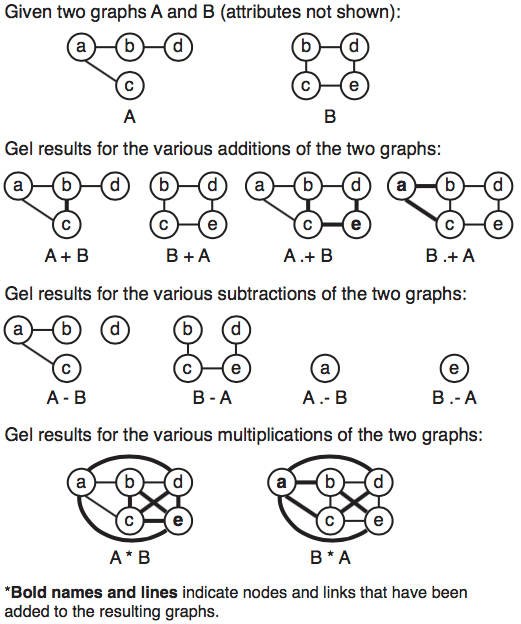# Graph Mathematics

One of the strengths of Gel is its set of graph mathematics. Each graph is treated as a unit that can be added, subtracted, or otherwise combined with each other. Gel takes care of attribute conflict and merging.

For a more intuitive introduction, take a look at the visual summary of graph mathematics section below. To understand graph mathematics from a tutorial, see section two. For the rigorous theoreticians among you, see section three.

Outline

• A visual summary of graph mathematics
• A gel tutorial demonstrating graph mathematics
• A theoretical explaination of graph mathematics

## A visual summary of graph mathematics

The following figure provides a visual of graph mathematics on two starting graphs: $G_A$ and $G_B$. New nodes and links from the right operand are shown in bold. Graph operations have dotted and non-dotted versions depending on node-centric or link-centric operations.## A Gel tutorial demonstrating graph mathematics

Run the following to create two graphs.

node(string id, int count) ntA;
graph(ntA,ltA) A = {("a",1)[0.4]("b",2)[0.4]("d",4), a[0.8]("c",3)};

node(string id, string tag) ntB;
graph(ntB,ltB) B={("b","g")[0.3]("d","m")[0.3]("e","c"), b[0.2]("c","g")[0.2]d};


Layout the graphs. Switch to the red and blue graph example

layout(A,"circle");
layout(B,"circle");
/* right click each panel to run force-directed and then right click to stop */

foreach node in A set _r=1,_text=name;
foreach link in A set _r=1,_text=weight;
foreach node in B set _b=1,_text=name;
foreach link in B set _b=1,_text=weight;


Try some graph mathematics.

/* This will save the results */
node(ntA,ntB) ntC;
graph(ntC,ltC) result;

/* Double click result in Data Panel and show in Graph Canvas panel */
/* Then try the following graph mathematics
result=A+B;
result=A.+B;
result=A-B;
result=A.-B;

/* Intersection of two graphs */
result=A & B;
result=A .& B;

/* Complete graph */
result=A * A;

/* Inverse graph */
result=A*A-A;


## A theoretical explanation of graph mathematics

A graph is defined as a set of nodes ($V$) and links ($E$) where a node represents an entity and a link represents a relationship between a pair of entities.

$G=\{V,E\} \quad \text{where} \quad V=\{v_1,v_2,v_3,...,v_n\} \quad \text{and} \quad \quad E\subset \{(v_i,v_j)|v_i,v_j \in V\}$

In practice, graphs have additional annotations called attributes. Currently, Gel provides four basic data primitives string, int, float and double as well as aggregate data types node ($V_{attr}$), link ($E_{attr}$) and graph.

$V_{attr}=\{a_1,a_2,a_3,...,a_{m1}|type(a_i)\in \{int, float, double, string\}\}$

$E_{attr}=\{a_1,a_2,a_3,...,a_{m2}|type(a_i)\in \{int, float, double, string\}\}$

Graph mathematics allow graph level operations. When two graphs are combined, node and link attributes must be combined in an intuitive manner. What happens when node and link attribute conflict occurs. The graph on the left (left operand) values take precedence. The exception to this is if the left operand has default values. Then the new data from the right will be incorporated into the new resulting graph.

Suppose you have two graphs $G_A$ and $G_B$:

$G_A=\{V_A,E_A\} \quad \text{and} \quad G_B=\{V_B,E_B\}$

Part of the confusion of combining and subsetting multiple graphs is what to do with the nodes and links. Gel provides dotted and non-dotted mathematics to specify node-centric or link-centric operations.

In order to merge links and nodes in two graphs use the dotted addition operator.

$G_A\ .+G_B=\{V_A \cup V_B, E_A \cup V_B\}$

However, if you are only concerned with nodes in $G_A$ such as a set of important genes or locations and only want to overlay new links from $G_B$, use the non-dotted addition operator.

$G_A +G_B=\{V_A, E_A \cup \{(v_i,v_j)|v_i,v_j \in V_A, (v_i,v_j) \in E_B\}\}$

In a similar fashion, the subtraction operator has a dotted and non-dottted version.

$G_A\ .-G_B=\{V_A \setminus V_B, E_A \setminus V_B\}$

$G_A -G_B=\{V_A, E_A \setminus V_B\}$

The intersection of two graphs also have dotted and non-dotted versions.

$G_A .\& G_B=\{V_A \cap V_B, E_A \cap E_B \}$

$G_A \& G_B=\{V_A, E_A \cap E_B \}$